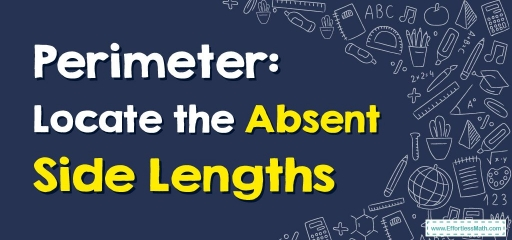# Perimeter: Locate the Absent Side Lengths

As you know, if you do not have the length of a polygon, you can use the perimeter to determine the length of the missing side. but how? This is what we are going to teach you in this article.A perimeter of a shape is described as the complete distance around a shape. It’s the outline’s length or the boundary of any $$2$$-dimensional geometric shape.

A perimeter of various figures could be identical in measurement dependent on their dimensions. For instance, think of a triangle made with a wire consisting of length $$L$$. The identical wire could be reprocessed into a square since all the sides are identical in length.

## How to Locate the Perimeter?

A perimeter is the measurement of length contained by the boundary of a shape. Therefore, the perimeter’s units are identical to the units of length. You can state that a perimeter is $$1$$-dimensional.

Consequently, you can measure it in centimeters, meters, kilometers, etc. A few additional units for doing a measurement of an accepted internationally are miles, yards, feet, and inches.

Perimeter = Amount of all the sides

If one side’s length is not there, employing perimeter you can locate the missing side’s length.

Step one: We are aware perimeters are the length around shapes. Thus, firstly add all sides that are known.

Step two: Subtract the quantity of the sides that are known from a perimeter to locate the missing side’s length.

Note: If you know a rectangle’s perimeter, as well as at least one side’s length, it’s possible to locate the additional $$3$$ sides yourself!

### Perimeter: Locate the Absent Side Lengths –Example 1:

Find the missing side of the shape.

Perimeter $$=48. x=$$___

Solution:

The perimeter of a square $$= 4 × side →$$ $$4 × x= 48 →$$ $$x=\frac{48}{4} = 12$$ $$→ x=12$$

### Perimeter: Locate the Absent Side Lengths –Example 2:

Find the missing side of the shape.

Perimeter $$=50. x=$$___

Solution:

The perimeter of a rectangle $$= 2 × (l + b)→$$ $$2× (14+x)= 50 →28+2x=50 →2x=22 →$$ $$x=\frac{22}{2} = 11$$ $$→ x=11$$

## Exercises for Perimeter: Locate the Absent Side Lengths

Find the missing side of each shape.

1) Perimeter $$=88. x=$$___

2) Perimeter $$=42. x=$$___

1. $$\color{blue}{22}$$
2. $$\color{blue}{ 8}$$

### What people say about "Perimeter: Locate the Absent Side Lengths - Effortless Math: We Help Students Learn to LOVE Mathematics"?

No one replied yet.

X
30% OFF

Limited time only!

Save Over 30%

SAVE $5 It was$16.99 now it is \$11.99